Published: 24 September 2018

# Position error estimation of the underwater coordinate measurement machine using artificial neural network

Soji Shimono1
Yuki Hagino2
Uichi Nishizawa3
Shigeki Toyama4
1Q.I Incorporated, Yokohama, Japan
1, 2, 3, 4Tokyo University of Agriculture and Technology, Tokyo, Japan
Corresponding Author:
Soji Shimono
Views 95

#### Abstract

Position error of the underwater coordinate measurement machine (UWCMM) is estimated using an artificial neural network (ANN). The UWCMM has a multi joint serial link and the position of an underwater robot attached to the tip is obtained from kinematics of the link. However, the inaccuracy of the kinematics model creates a position error. In order to improve measurement accuracy, an ANN model which estimate position error of the kinematics of the UWCMM using joint angles data is constructed. The ANN model was trained by 462 data and verified by 42 data. The error estimation result of the trained ANN reduces maximally 66.5 % of the average of the position error of the verification data.

## 1. Introduction

Underwater robots are necessary for underwater observation such as deep sea exploration, sea floor resource development, and inspection of the underwater facility such as dam. Because human activities are limited in the underwater environment. The positioning is one of the important technologies for underwater robots. Unlike the atmosphere, in the underwater, the global navigation satellite system (GNSS) is not available because the water attenuates the electromagnetic wave from the satellite of the GNSS. Therefore, usually, the acoustic positioning system and the inertial navigation system are used for the underwater positioning. As a different approach from these devices, underwater coordinate measurement machine (UWCMM) is proposed. The UWCMM consist a multi joint serial link and an underwater robot. The underwater robot is connected to the serial link inserted into the water from a base point which is set on the water surface (Fig. 1). The joint angles of the serial link is measured by itself and the related position between the underwater robot and the base point can be obtained. And the position of the base point is obtained from the GNSS. This system has following advantages: unlike the inertial navigation system, position drift with time advance is not appear; proper underwater sound velocity information at the target area which is needed for the acoustic positioning is not necessary, and no position error due to the multi pass of the sound wave near the underwater structure.

In the previous study about the UWCMM, developed an experimental model is reported . And its calibration of the kinematics based on the Denavit-Hartenberg (DH) notation and evaluation of the position accuracy are reported . In this study, in order to improve position accuracy, a position error estimation model based on artificial neural network (ANN) is constructed. The kinematics based on the DH notation and its calibration does not take into account the non-geometric factor such as backlash, bending. Thus, the position error due to these factors is not reduced by calibration of the DH parameters. Therefore, an ANN model which estimate position error of the kinematics based on DH method using joint angles information is constructed. In this paper, the developed test model and implemented calibration are overviewed and the constructed error estimation model based on ANN and its error estimation results are reported.

Fig. 1Concept of the UWCMM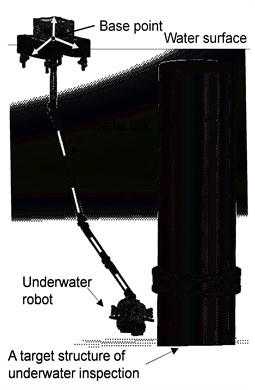Fig. 2Experimental model of the UWCMM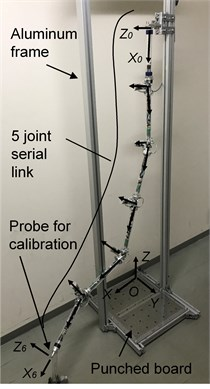## 2. Experimental model and calibration

The developed experimental model of the UWCMM is shown in Fig. 2. Although the tip of the link is connected to the underwater robot during the underwater observation, in Fig. 2, a steel ball probe is attached for position measurement experiment. The experimental model has five number of single axis joint, and each joint has an absolute rotary encoder which has 18 bit resolution. Each link involves a micro controller and it read the joint angle from the encoder and transmit the joint angle to a data logger at the water surface. The position of the probe is calculated by the kinematics based on the DH notation using joint angles. The design values of the DH parameters of the multi joint serial link is shown in Table 1. The parameters represent movement and rotation between the coordinate systems. The $a$ indicates movement along the $x$ axis, the $d$ indicates movement along the $z$ axis, $\alpha$ indicates rotation around the $x$ axis, and the θ indicates rotation around the $z$ axis, respectively.

Usually, geometric parameters of the actual machine have difference from the designed value due to the machining error, and the assemble error. Thus, calibration of the DH parameters is implemented. Detail of the calibration method is described in the . The basic idea of the calibration is as follows: the tip position vector is obtained from the kinematics using joint angles and DH parameters; the relation equation between small displacement of the tip position and small displacement of the DH parameters is obtained from the Jacob matrix about the DH parameter of the position vector; the correction value of the parameters is calculated by the inverse matrix of the Jacob matrix of the relation equation and the position error.

To conduct the calibration, several pieces of data is required. The data consist the position error and the joint angles at that time. It is obtained from a position measurement experiment using a punched board that set on the aluminum frame (Fig. 2). The board has 42 holes whose position are known. The probe attached to the tip of the link is set on the hole and each joint angle is recorded at that time. The position error is the difference between position of the hole and the probe position calculated by the kinematics of the multi joint serial link.

For the calibration and the ANN training, 504 data were obtained by the position measurement experiment. The experiment was conducted with two postures of the multi joint serial link. The one was the angle of the tip joint was rotate positive direction and the other is the angle was rotate negative direction. All holes were measured six times with two posture of the serial link respectively. The experiment was conducted underwater. The experimental model was attached to the aluminum frame. The frame was sinking to the bottom of a water tank approximately 2 m deep.

Table 1DH parameter of experimental model

 Coordinate system $a$ [mm] $d$ [mm] $\alpha$ [degree] $\theta$ [degree] 0 → 1 284 0 0 ${\theta }_{1}$ 1 → 2 330 0 90 ${\theta }_{2}$ 2 → 3 330 0 –90 ${\theta }_{3}$ 3 → 4 330 0 90 ${\theta }_{4}$ 4 → 5 330 0 –90 ${\theta }_{5}$ 5 → 6 339 – – –

## 3. Error estimation using ANN

The implemented calibration is not considering the non-geometric factor and it produce position error. To improve positioning accuracy, an error estimation model based on ANN is constructed. As a deployment of ANN to the multi joint mechanism, there are kinematics approximation for the manipulator [3-5], and position error estimation using three joint angles of the manipulator which has 6 degree of freedom . In this paper, a position error estimation model for the UWCMM based on ANN which estimate position error from five joint angles information is constructed.

The model is implemented with the Keras and the TensorFlow that are software library for the machine learning. The network of the constructed model is shown in Fig. 3. The input layer consist five node and it is input each joint angle which is normalized. The output layer consists three neurons that express the position error in $x$, $y$, and $z$ direction respectively. The hidden layer has four layers and each layer consist 175 neurons. The initializer for the input layer neurons is set to the lecun_uniform method , and the others initialized with the default method of the Keras. The activation function at the output layer is the linear function to represent position error. And the other layer uses the rectified linear unit (ReLU) function [8, 9] as the activation function. The evaluation function for training is selected the mean square error. The optimizer is selected the Adam method , and the beta1 is 0.9, the beta2 is 0.999 and the learning late is 0.001. The batch size is 42 that is the same to the number of holes on the punched board.

Fig. 3Network structure of used ANN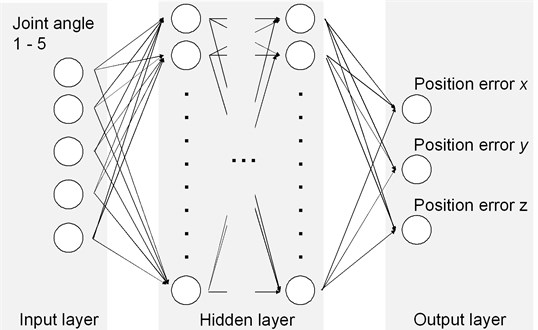## 4. Results of error estimation

When the data of the 42 holes are defined as one dataset, the 504 data that was obtained the position measurement experiment consists 12 datasets. One dataset was used as the verification data and the other datasets were used for the calibration and the training of the ANN. Training of the ANN was repeated up to 100 times after improvement of the result of the evaluation function was no longer observed.

A result of the position error estimation by a trained ANN at the $x$, $y$ direction is shown in Fig. 4, and Fig. 5, respectively. The 12th dataset was selected as the verification data at this result. The trained ANN well predict the target error that is the position error of the kinematics. The averageand the maximum value of the position error about the 42 holes after the calibration and compensated result by the trained ANNs are shown in Fig. 6, and Fig. 7. These figures show the results when each dataset is selected as verification data. Training of the ANN and the error estimation using the trained ANN was 20 times conducted at each verification dataset. The average of the compensated error is the average of the result of each trained ANN. Although the rate of the effect varies, Fig. 6, and Fig. 7 indicate that the trained ANNs reduce the average of the position error and the maximum error in each dataset. For the maximum case, the average of the position error is 66.5 % reduced by the trained ANN. The result shows that the constructed ANN model is effective for improvement of the positioning accuracy.

Fig. 4Error estimation at x direction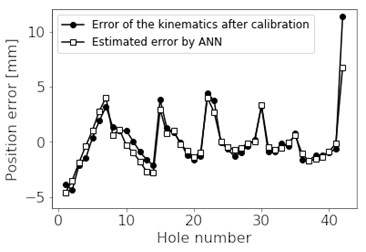Fig. 5Error estimation at y direction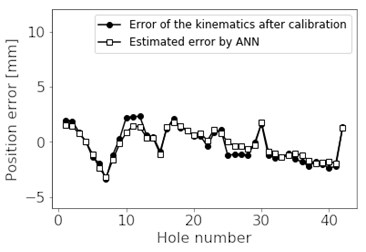Fig. 6Average of position error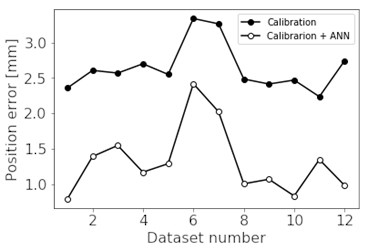Fig. 7Maximum position error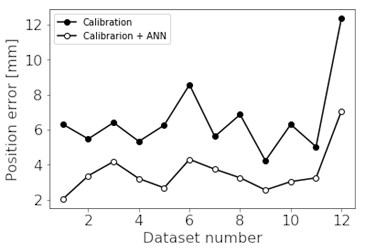## 5. Conclusions

A position error estimation model based on ANN is constructed to improve positioning accuracy of the UWCMM which consist of multi joint serial link. The ANN was trained with 462 data that consist of position error of the kinematics and joint angles of the serial link. And the trained ANN was validated with 42 data. It is confirmed that the position error of the calibrated experimental model of the UWCMM is reduced by the ANN based error estimation model. In the maximum case, 66.5 % of average of the position error is reduced.